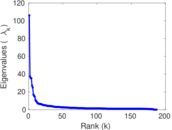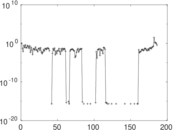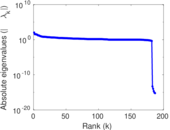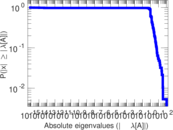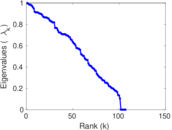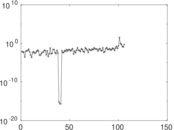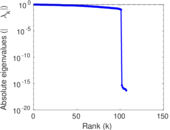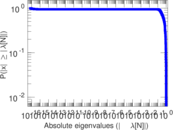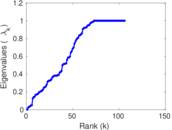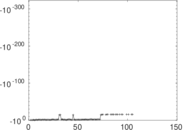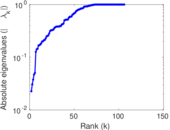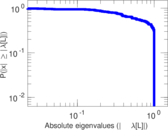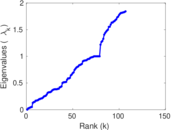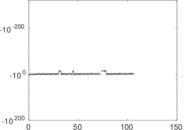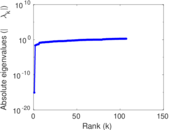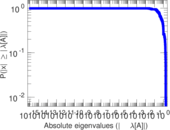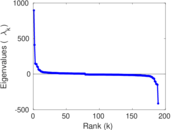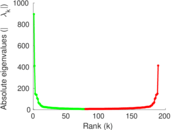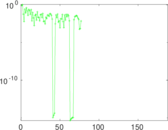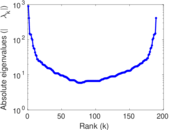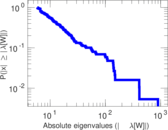# Wikiquote edits (br)

This is the bipartite edit network of the Breton Wikiquote. It contains users and pages from the Breton Wikiquote, connected by edit events. Each edge represents an edit. The dataset includes the timestamp of each edit.

 Code `qbr` Internal name `edit-brwikiquote` Name Wikiquote edits (br) Data source http://dumps.wikimedia.org/ AvailabilityDataset is available for download Consistency checkDataset passed all tests Category Authorship network Dataset timestamp 2017-10-20 Node meaning User, article Edge meaning Edit Network formatBipartite, undirected Edge typeUnweighted, multiple edges Temporal dataEdges are annotated with timestamps

## Statistics

 Size n = 906 Left size n1 = 189 Right size n2 = 717 Volume m = 2,625 Unique edge count m̿ = 1,440 Wedge count s = 56,506 Claw count z = 2,808,087 Cross count x = 126,564,947 Square count q = 23,761 4-Tour count T4 = 420,864 Maximum degree dmax = 679 Maximum left degree d1max = 679 Maximum right degree d2max = 98 Average degree d = 5.794 70 Average left degree d1 = 13.888 9 Average right degree d2 = 3.661 09 Fill p = 0.010 626 3 Average edge multiplicity m̃ = 1.822 92 Size of LCC N = 677 Diameter δ = 14 50-Percentile effective diameter δ0.5 = 3.486 08 90-Percentile effective diameter δ0.9 = 6.010 96 Median distance δM = 4 Mean distance δm = 4.199 08 Gini coefficient G = 0.754 747 Balanced inequality ratio P = 0.186 095 Left balanced inequality ratio P1 = 0.151 619 Right balanced inequality ratio P2 = 0.245 714 Relative edge distribution entropy Her = 0.836 625 Power law exponent γ = 3.020 51 Tail power law exponent γt = 2.091 00 Tail power law exponent with p γ3 = 2.091 00 p-value p = 0.010 000 0 Left tail power law exponent with p γ3,1 = 1.741 00 Left p-value p1 = 0.344 000 Right tail power law exponent with p γ3,2 = 2.271 00 Right p-value p2 = 0.000 00 Degree assortativity ρ = −0.203 619 Degree assortativity p-value pρ = 6.095 76 × 10−15 Spectral norm α = 106.011 Algebraic connectivity a = 0.022 540 9 Spectral separation |λ1[A] / λ2[A]| = 2.839 11 Controllability C = 531 Relative controllability Cr = 0.593 296

## Plots

### Fruchterman–Reingold graph drawing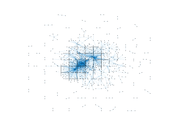### Degree distribution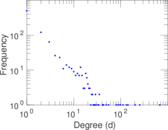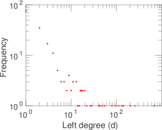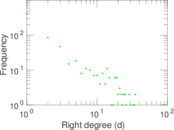### Cumulative degree distribution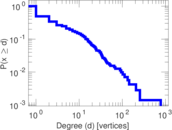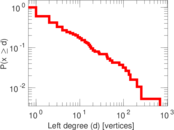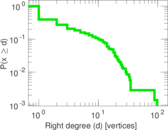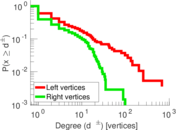### Lorenz curve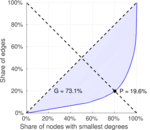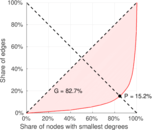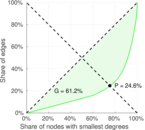### Spectral distribution of the adjacency matrix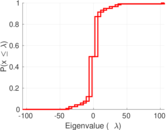### Spectral distribution of the normalized adjacency matrix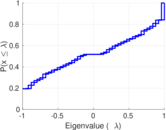### Spectral distribution of the Laplacian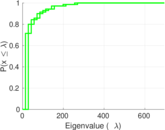### Spectral graph drawing based on the adjacency matrix### Spectral graph drawing based on the Laplacian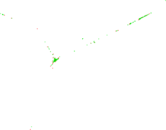### Spectral graph drawing based on the normalized adjacency matrix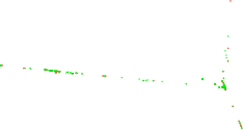### Degree assortativity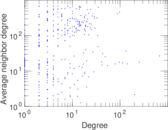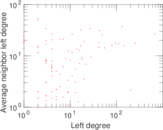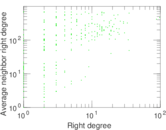### Zipf plot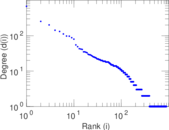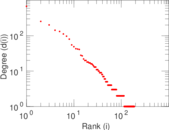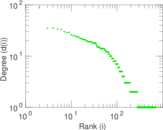### Hop distribution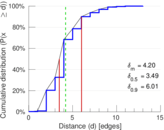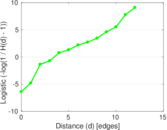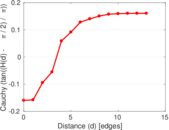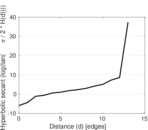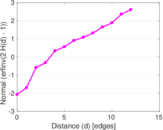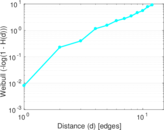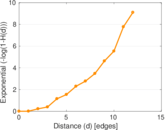### Double Laplacian graph drawing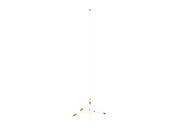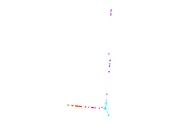### Delaunay graph drawing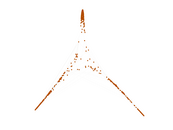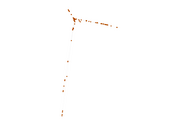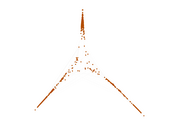### Edge weight/multiplicity distribution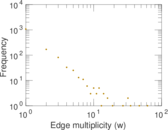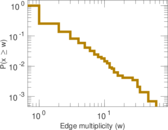### Temporal distribution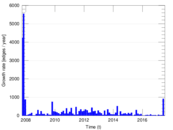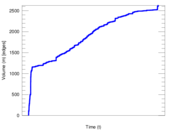### Temporal hop distribution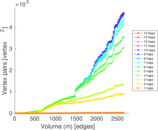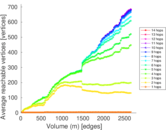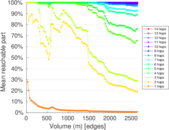### Diameter/density evolution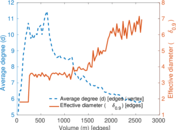### Matrix decompositions plots